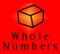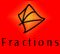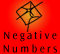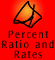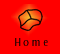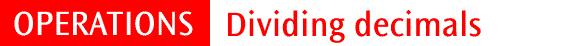| Dividing a decimal number by a whole number | Dividing a whole number by a decimal number | Dividing a decimal number by a decimal | Performing division with decimal numbers | Links to fraction operations |
| Quick Quiz | Mixed operations quiz | FAQs |

There are three main types of division problems involving decimals:

• dividing a whole number by a decimal number (24 ÷ 0.3)
• dividing a decimal number by a whole number (2.4 ÷ 3)
• dividing a decimal number by a decimal number (2.4 ÷ 0.3)

As with whole number division, the meaning of division problems can be explained by thinking of them as quotition or partition problems, depending on the numbers involved.

One of the most important factors to remember in the division of decimals is place value, that each column to the left is ten times larger than that to the right and thus every column to the right is ten times smaller than that to the left.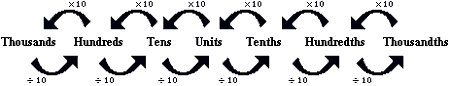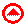Dividing a decimal number by a whole number

In this example we will use partition division to work out the problem.

Example 1:

I have 24 cakes to share between three people. How many cakes will each person receive?

24 ÷ 3 = 8

 Working Thinking I need to share 24 items between three people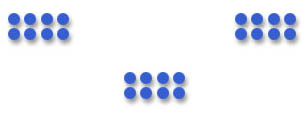Deal out 24 items to 3 people.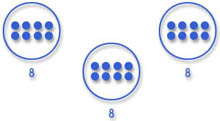Count the number of items in each group. There are 8 items in each groups

We can apply these ideas to division of decimals.

I have 2.4 cakes to share between three people. How much will each person receive?

2.4 ÷ 3 = 0.8

 Working Thinking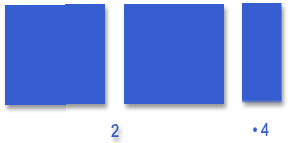2.4 shared between three people. Each person will receive less than a whole cake because we started with less than three cakes.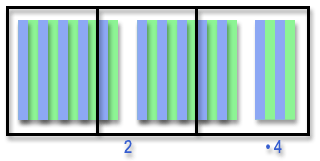Divide the whole cakes into ten tenths each. So 2.4 cakes are now thought of as 24 tenths of cake. Then split equally into three groups.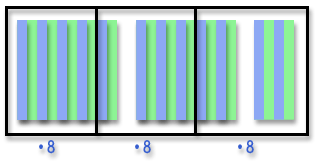Count up each group. There are eight tenths of a cake in each group. Therefore each person receives 0.8 cakes.

If we look at the relationship between these two problems we can see a similar relationship as when we divided a whole number by a decimal number.

 24 ÷ 3 = 8 2.4 ÷ 3 = 0.8 The amount to be divided is 10 times smaller. The number of people to share between remains the same The answer is ten times smaller.

The following chart allows you to see the pattern.

 24 ÷ 3 = 8 24 ones divided by 3 ones equals 8 ones 2.4 ÷ 3 = 0.8 24 tenths divided by 3 ones equals 8 tenths 0.24 ÷ 3 = 0.08 24 hundredths divided by 3 ones equals 8 hundredths 0.024 ÷ 3 = 0.008 24 thousandths divided by 3 ones equals 8 thousandths 0.0024 ÷ 3 = 0.0008 24 ten thousandths divided by 3 ones equals 8 ten thousandthsDividing a whole number by a decimal number

It is usually best to think of these problems using quotition division.

I am going to bake 24 cakes for my party. Each friend will eat 3 cakes each. How many friends can I invite?

Example 2: 24 ÷ 3 = 8

 Working Thinking How many groups of 3 in 24?Each group has to have three in itCount the number of groups. There are 8 groups

The same idea applies in using decimals.

Example 3: How many groups of 0.3 in 24?

24 ÷ 0.3 = 80

I am baking 24 cakes for my party and each person will have 0.3 of a cake each. How many people can I invite?

We know that in this case the amount of cake each person receives is smaller than in the first question, because 0.3 is only a tenth of 3. Therefore we can invite ten times as many people.

This is ten times more than the answer in the first question, 24 ÷ 3, so the answer is 80.

 24 ÷ 3 = 8 24 ÷ 0.3 = 80 0.3 is one tenth of 3. The amount each person received has decreased. The answer, 80, is ten times as large as 8. Therefore the number of people we can feed increases.

When the divisor becomes ten times smaller the answer becomes ten times larger.

The following chart allows you to see this pattern:

 24 ÷ 3 = 8 24 ones divided by 3 ones equals 8 ones 24 ÷ 0.3 = 80 24 ones divided by 3 tenths equals 8 tens 24 ÷ 0.03 = 800 24 ones divided by 3 hundredths equals 8 hundreds 24 ÷ 0.003 = 8 000 24 ones divided by 3 thousandths equals 8 thousands 24 ÷ 0.0003 = 80 000 24 ones divided by 3 ten thousandths equals 8 ten thousands

Knowledge of the basic division facts in this case allows you to perform division of decimals by adjusting the place value of the numbers.Dividing a decimal number by a decimal number

The most difficult divisions involve dividing decimals by decimals. Take for example 2.4 ÷ 0.03. How many groups of 3 hundredths in 2.4? We know from previous examples with 24 ÷ 3 that the answer will contain an eight.

We can estimate that the answer will be quite large because we are finding how many 3 hundredths are in 2.4. We know that 2.4 is the same as 240 hundredths, therefore we can ask how many 3 hundredths are in 240 hundredths.

240 divided by 3 is 80. There are 80 groups of 3 hundredths in 240 hundredths.

2.4 ÷ 0.03 = 80

An overall picture of the relationship between the place value of the numbers being divided and the answer can be summarised in the following chart. As we go across the chart the number to be divided increases by a factor of 10, so the answers increase by a factor of 10. As we go down the chart the divisors increase by a factor of 10 so the answers decrease by a factor of 10. Remember that the order of numbers is important in division, for example, 0.24 ÷ 3 is not the same as 3 ÷ 0.24.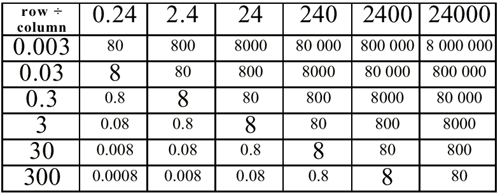Performing division with decimal numbers

Dividing by a whole number

Dividing a decimal by a whole number can be carried out with only a slight variation of the usual algorithm.

Example 1: Dividing by a whole number - interpreted as partition division

0.125 ÷ 5 = 0.025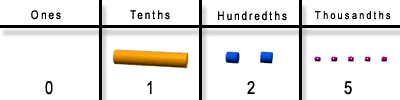0.125, shared between five? I set up 0.125 using LAB and I need to divide this into 5 equal groups. I start with the tenths column. One tenth, divided by five? I can't do this evenly so I will need to rename the one tenth.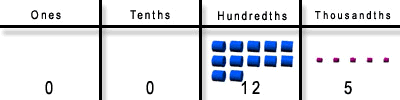I know that one tenth is the same as 10 hundredths so I will rename it as ten hundredths. I now have twelve hundredths.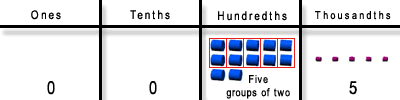Twelve hundredths shared into 5 equal groups. Two hundredths can be allocated to each group, and I still have two hundredths remaining.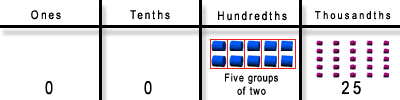Again, I rename the two hundredths as twenty thousandths. I add the twenty thousandths to the five thousandths and now have to share twenty-five thousandths into 5 equal groups.Twenty-five shared amongst 5 groups is five in each group and there are no thousandths left. (If there were some left over, I would rename them as ten-thousandths.) Each group therefore contains 2 hundredths and 5 thousandths. There are 5 groups of 0.025 in 0.125.

Understanding that division is the inverse operation of multiplication is also useful in checking answers.

If we multiply 0.025 x 5 the answer is 0.125.

Example 2: Dividing by a whole number using long division.
(NOTE: Short division would be the method usually used for a question like this)

2.504 ÷ 8 = 0.313

Dividing by a decimal number

Dividing by a decimal number can be carried out by changing the problem (but not the answer!) so that it is division by a whole number, which we know how to do.

For example, all these divisions have the same answer. In every case, the first number is four times as big as the second. 8 is four times 2, 8 thousand is four times 2 thousand, 8 millionths is four times 2 millionths.

8 ÷ 2 = 4

80 ÷ 20 = 4

8000 ÷ 2000 = 4

0.8 ÷ 0.2 = 4

0.000008 ÷ 0.000002 = 4

Example 3: Dividing by a decimal by changing to whole numbers.

0.08 ÷ 0.02 = 4

Example 4: Dividing by a decimal.

2.628 ÷ 0.12

Example 5: Dividing by a decimal (needing to add additional decimal places)

1.257 ÷ 0.15 = 8.38

In Example 5, the division reached no remainder after one zero had been added to 1.257, making it 1.2570. In other cases, more zeros will be required.

In some cases, the division will never reach no remainder and you need to make a decision to stop when sufficient accuracy has been attained. For example 1÷ 6 gives 0.166666 repeating. The answer can be rounded to a desired accuracy for practical purposes.Operations with decimals can be performed by writing them in their common fraction form. The same answer will be obtained, although the algorithm is different.

For example, 45 ÷ 0.9.

We know that the answer will be a little bit larger than 45 because 0.9 is less than one.

Decimal algorithm

To divide by the decimal, notice that 45 ÷ 0.9 has the same answer as 450 ÷ 9, which is 50.

Fraction algorithm

We can write 45 as a fraction: 45/1, and 0.9 is nine tenths so we can write it as 9/10.

45/1 ÷ 9/10. To divide by 9/10, we multiply by its inverse. Invert the fraction to get 10/9 and carry out multiplication.

45/1 x 10/9 = (45x10)/(1x9) = 450/9 = 50Division Quiz

1. Given that 79475 ÷ 5 = 15895, find:

 (a) 794.75 ÷ 5 (b) 79.475 ÷ 0.5 (c) 7.9475 ÷ 0.05 (d) 7.9475 ÷ 0.005

 2 45 ÷ 0.5 3 6.864 ÷ 2.2

Extra questions:

1. 14.606 ÷ 0.4

2. Find the quotient when 28.941 is divided by 0.03

3. Evaluate 35.4 ÷ 60

4. Sean has a 57.6cm length of ribbon. He wishes to cut 12 equal sized pieces of ribbon from this. What will the length of each piece be?

5. How many doses of medicine are there in a bottle containing 18.4 millilitres if each dose is 0.8 millilitres?

6. \$328.86 is to be shared between 9 people. How much will each person receive?Mixed operations quiz

1. What is the sum of the greatest and the least of the numbers 0.707, 0.72, 0.07 and 0.207?

2. Which one of the following is the smallest decimal: 0.7031, 0.731, 0.7003, 0.701?

3. Round 89.765 to the nearest tenth.

4. Nine cyclists each cycle 24.3 km. What is the combined distance traveled by the cyclists?

5. What is the largest number that can be produced using any of the numbers 4.12, 4.8, 0.8 or 1.2 once only in the following number sentence.

÷
-
=

6. Find the product of the sum and difference of 13.17 and 12.19

7. A loaf of bread cost \$2.33 and a carton of milk cost \$1.85. Find the cost of 4 loaves of bread and two cartons of milk.

8. Round 45.32648 to three decimal places.

9. Find a number that lies between 7.5 and 8.5

10. Write the numeral for seventeen ones + thirteen tenths + one hundred and twenty seven hundredths.

11. If 394.185 ÷ 16.5 = 23.89, find 3.94185 ÷ 1650

12. How much more is the product of 5.2 and 1.6 than the quotient obtained when the larger number is divided by the smaller number?

13. Mr. Benson bought 0.6 kilograms of cheese at \$4.25 a kilogram. How much did he pay for the piece of cheese?

14. A ship carried 12 containers each weighing 4.245 tonnes and 6 containers whose combined weight was 23.976 tonnes less than the combined weight of the 12 containers. What was the total weight of these18 containers?

15. When Alice attended a mathematics lesson in Magic Land, she found that A*B = (A x A) - (B x B). What would be the value of 0.2*0.02 in Magic Land?

16. Mrs. Ng wants to buy some lawn seed. She reads on the packet that 100square metres of lawn require 3.5 kg of lawn seed. Her lawn occupies 960 square metres.

(a) How much lawn seed does she need to buy?

(b) If lawn seed is sold in 4 kg packets, how many packets will she need to purchase?

17. A certain number is multiplied by 4 and when 2.76 is subtracted from this answer the result is 0.64. What is the number?

18. Tim traveled 4.35 km. This was 0.19 km more than Bill traveled. The total distance traveled by Barbara, Cathy, Bill and Tim was 14.67 km. If Barbara and Cathy traveled an equal distance, how far did each of the girls travel?

19.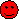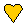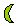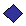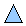The above shapes each represent a different tenths value. The sum of the rows and columns are given in the table below. Find the value of each shape.0.81.00.8 0.6 1.0 1.0

20. The sum of two numbers is 4.2 and their difference is 0.6. What are the two numbers?FAQs

 What are quotition and partition problems? Partition involves sharing between a given number. For example, 24 ÷ 3. We are sharing 24 cakes between 3 people. How many cakes will each person receive? Quotition involves dividing a number into a given quota. For example, 24 ÷ 3. Our quota is three. This can be thought of as "How many groups of 3 are there in 24?"

 What do we mean by inverse operations? An inverse operation reverses or "undoes" the action of another operation. Addition and subtraction are inverse operations, and multiplication and division are inverse operations. If I add 17 to any number, then subtract 17, I get back to the number. If I subtract 17 from any number, then add 17, I get back to the number. If I multiply any number by 17, then divide by 17, I get back to the number. If I divide any number by 17, then multiply by 17, I get back to the number. These properties hold for all numbers not just 17 (except division by zero).